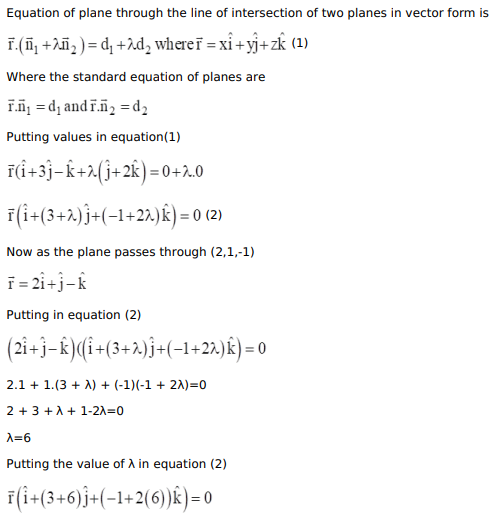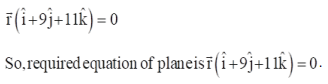# Find the vector equation of the plane through the intersection of the planesQuestion:

Find the vector equation of the plane through the intersection of the planes  $\overrightarrow{\mathrm{r}} \cdot(\hat{\mathrm{i}}+3 \hat{\mathrm{j}}-\hat{\mathrm{k}})=0$ and $\overrightarrow{\mathrm{r}} \cdot(\hat{\mathrm{j}}+2 \hat{\mathrm{k}})=0$. and passing through the point $(2,1,-1)$

Solution: